Next: Wind Driven Waves in Up: Waves in Incompressible Fluids Previous: Capillary Waves

# Capillary Waves at an Interface

Consider a layer of fluid of density, depth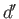, and uniform horizontal velocity, situated on top of a layer of another fluid of density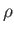, depth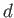, and uniform horizontal velocity. Suppose that the fluids are bounded from above and below by rigid horizontal planes. Let these planes be at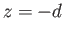and, and let the unperturbed interface between the two fluids be at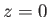. Suppose that the elevation of the perturbed interface is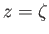, where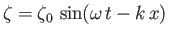. Finally, let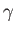be the surface tension of the interface. Equations (11.97), (11.98), and (11.118) yield the dispersion relation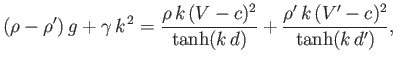(11.132)

which is a generalization of the dispersion relation (11.100) that takes surface tension into account. Here,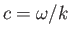is the phase velocity of a wave propagating along the interface.

For the case in which both fluids are at rest, and of infinite depth, the previous dispersion relation simplifies to give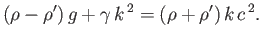(11.133)

Suppose that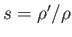is the specific gravity of the upper fluid with respect to the lower. In the case in which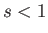(i.e., the upper fluid is lighter than the lower one), it is helpful to define(11.134)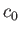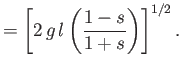(11.135)

It follows that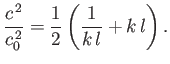(11.136)

Thus, we conclude that the phase velocity of a wave propagating along the interface between the two fluids achieves its minimum value,, when. Furthermore, waves of all wavelength are able to propagate along the interface (i.e.,for all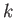). In the opposite case, in which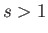(i.e., the upper fluid is heavier than the lower one), we can redefine the capillary length as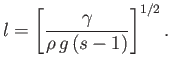(11.137)

The dispersion relation (11.133) then becomes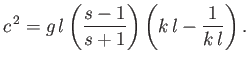(11.138)

It is apparent thatfor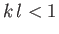, indicating instability of the interface for waves whose wavelengths exceed the critical value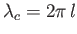. On the other hand, waves whose wavelengths are less than the critical value are stabilized by surface tension. This result is exemplified by the experiment in which water is retained by atmospheric pressure in an inverted glass whose mouth is closed by a gauze of fine mesh (the purpose of which is to put an upper limit on the wavelengths of waves that can exist at the interface.)Next: Wind Driven Waves in Up: Waves in Incompressible Fluids Previous: Capillary Waves
Richard Fitzpatrick 2016-03-31